# Linear Algebra A gentle introduction Linear Algebra has

• Slides: 29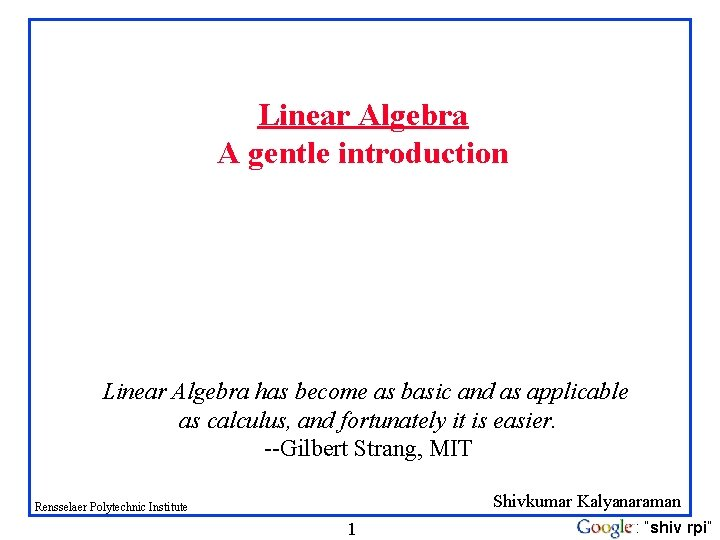Linear Algebra A gentle introduction Linear Algebra has become as basic and as applicable as calculus, and fortunately it is easier. --Gilbert Strang, MIT Shivkumar Kalyanaraman Rensselaer Polytechnic Institute 1 : “shiv rpi”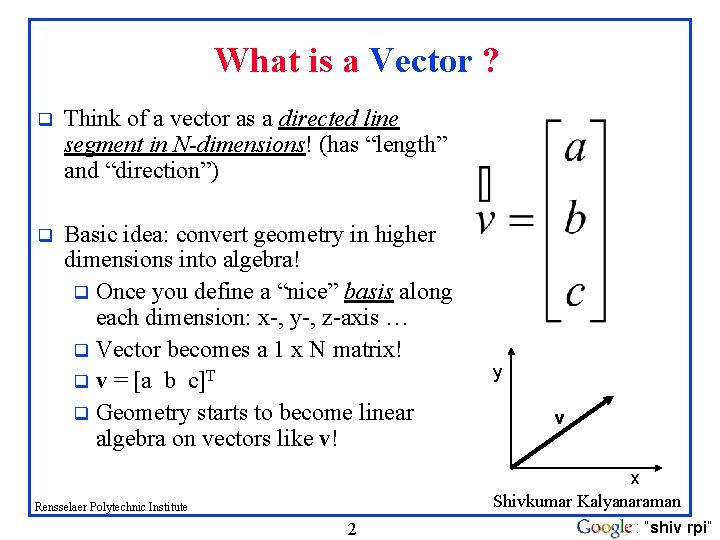What is a Vector ? q Think of a vector as a directed line segment in N-dimensions! (has “length” and “direction”) q Basic idea: convert geometry in higher dimensions into algebra! q Once you define a “nice” basis along each dimension: x-, y-, z-axis … q Vector becomes a 1 x N matrix! q v = [a b c]T q Geometry starts to become linear algebra on vectors like v! y v x Shivkumar Kalyanaraman Rensselaer Polytechnic Institute 2 : “shiv rpi”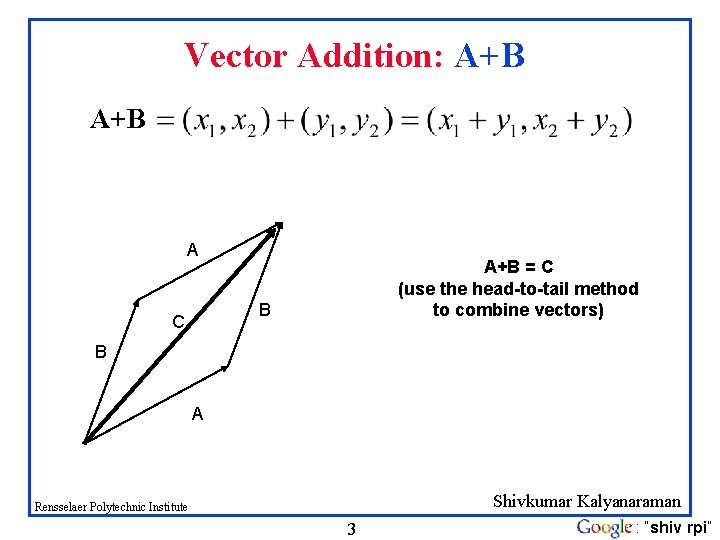Vector Addition: A+B A A+B = C (use the head-to-tail method to combine vectors) B C B A Shivkumar Kalyanaraman Rensselaer Polytechnic Institute 3 : “shiv rpi”Scalar Product: av av v Change only the length (“scaling”), but keep direction fixed. Sneak peek: matrix operation (Av) can change length, direction and also dimensionality! Shivkumar Kalyanaraman Rensselaer Polytechnic Institute 4 : “shiv rpi”Vectors: Dot Product Think of the dot product as a matrix multiplication The magnitude is the dot product of a vector with itself The dot product is also related to the angle between the two vectors Shivkumar Kalyanaraman Rensselaer Polytechnic Institute 5 : “shiv rpi”Inner (dot) Product: v. w or w. Tv v w The inner product is a SCALAR! If vectors v, w are “columns”, then dot product is w. Tv Shivkumar Kalyanaraman Rensselaer Polytechnic Institute 6 : “shiv rpi”Bases & Orthonormal Bases q Basis (or axes): frame of reference vs Basis: a space is totally defined by a set of vectors – any point is a linear combination of the basis Ortho-Normal: orthogonal + normal [Sneak peek: Orthogonal: dot product is zero Normal: magnitude is one ] Rensselaer Polytechnic Institute 7 Shivkumar Kalyanaraman : “shiv rpi”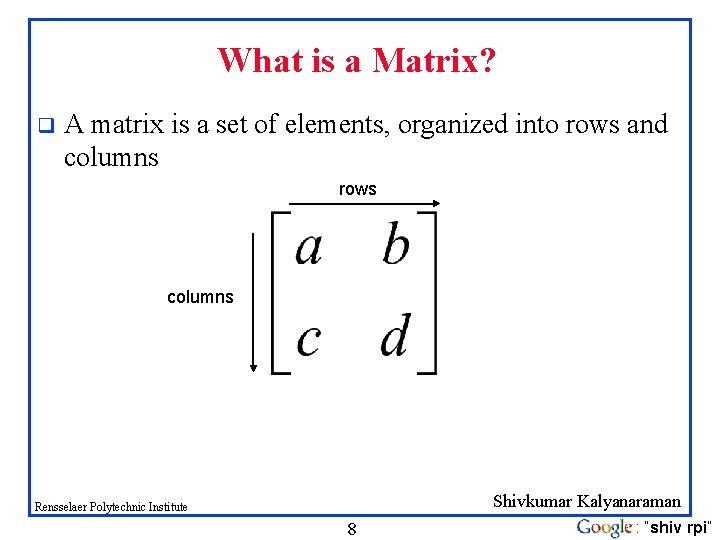What is a Matrix? q A matrix is a set of elements, organized into rows and columns rows columns Shivkumar Kalyanaraman Rensselaer Polytechnic Institute 8 : “shiv rpi”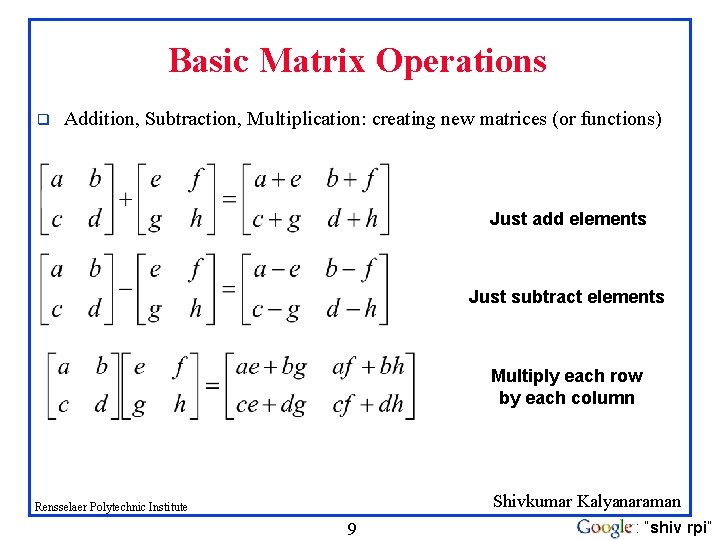Basic Matrix Operations q Addition, Subtraction, Multiplication: creating new matrices (or functions) Just add elements Just subtract elements Multiply each row by each column Shivkumar Kalyanaraman Rensselaer Polytechnic Institute 9 : “shiv rpi”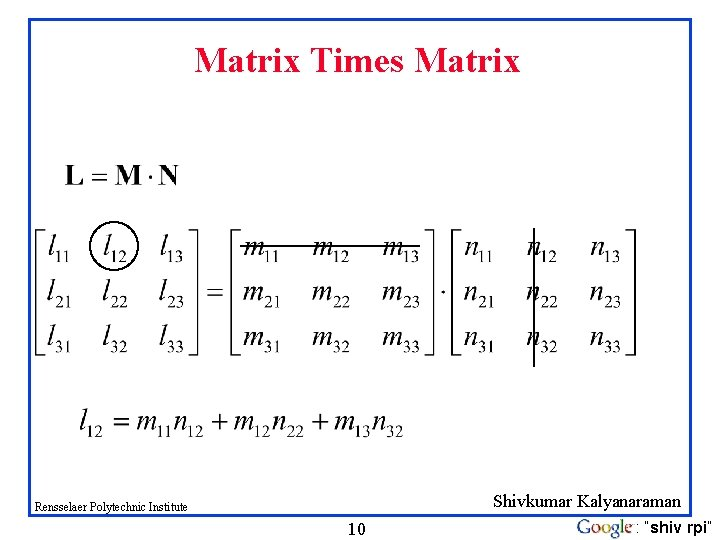Matrix Times Matrix Shivkumar Kalyanaraman Rensselaer Polytechnic Institute 10 : “shiv rpi”Multiplication q Is AB = BA? Maybe, but maybe not! q Matrix multiplication AB: apply transformation B first, and then again transform using A! Heads up: multiplication is NOT commutative! q q Note: If A and B both represent either pure “rotation” or “scaling” they can be interchanged (i. e. AB = BA) Shivkumar Kalyanaraman Rensselaer Polytechnic Institute 11 : “shiv rpi”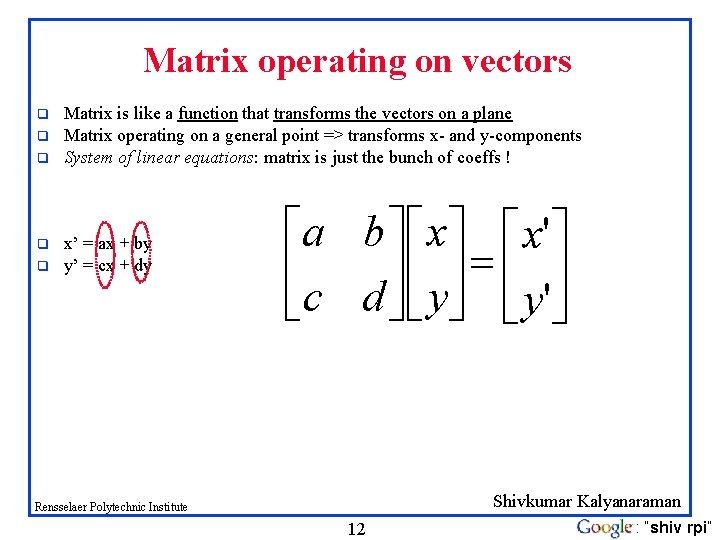Matrix operating on vectors q q q Matrix is like a function that transforms the vectors on a plane Matrix operating on a general point => transforms x- and y-components System of linear equations: matrix is just the bunch of coeffs ! x’ = ax + by y’ = cx + dy éa b ù é x'ù ê úê ú = ê ú ëc d û ë y'û Shivkumar Kalyanaraman Rensselaer Polytechnic Institute 12 : “shiv rpi”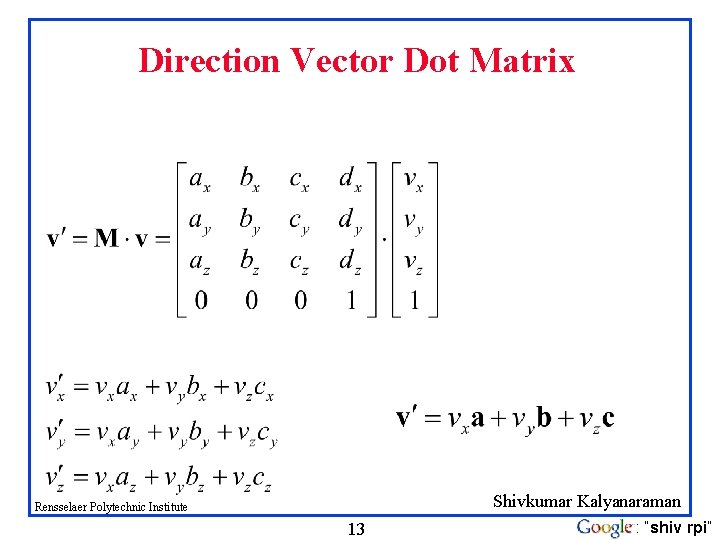Direction Vector Dot Matrix Shivkumar Kalyanaraman Rensselaer Polytechnic Institute 13 : “shiv rpi”Matrices: Scaling, Rotation, Identity q q q Pure scaling, no rotation => “diagonal matrix” (note: x-, y-axes could be scaled differently!) Pure rotation, no stretching => “orthogonal matrix” O Identity (“do nothing”) matrix = unit scaling, no rotation! r 1 0 0 r 2 [0, 1]T [0, r 2]T scaling [r 1, 0]T [1, 0]T cos -sin cos [0, 1]T rotation [-sin , cos ]T [cos , sin ]T [1, 0]T Shivkumar Kalyanaraman Rensselaer Polytechnic Institute 14 : “shiv rpi”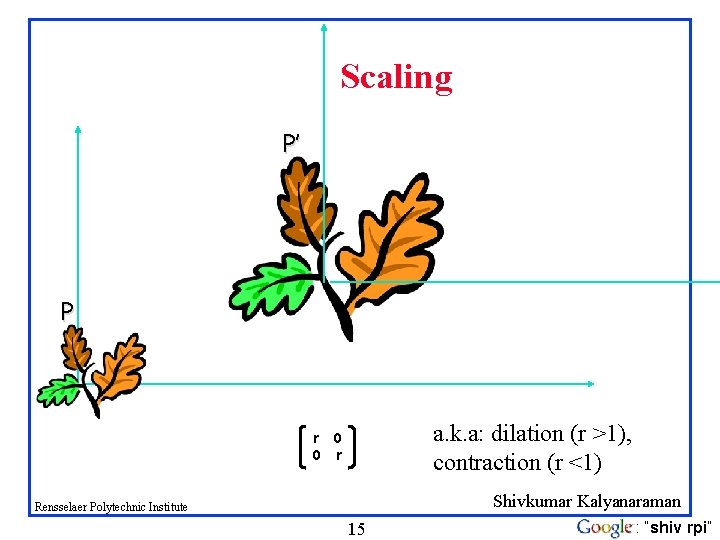Scaling P’ P a. k. a: dilation (r >1), contraction (r <1) r 0 0 r Shivkumar Kalyanaraman Rensselaer Polytechnic Institute 15 : “shiv rpi”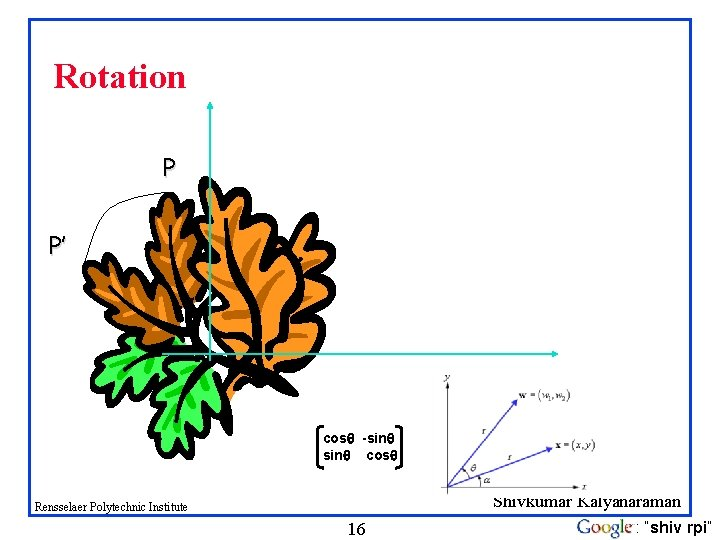Rotation P P’ cos -sin cos Shivkumar Kalyanaraman Rensselaer Polytechnic Institute 16 : “shiv rpi”2 D Translation P’ t P ty y P x Rensselaer Polytechnic Institute 17 P’ t tx Shivkumar Kalyanaraman : “shiv rpi”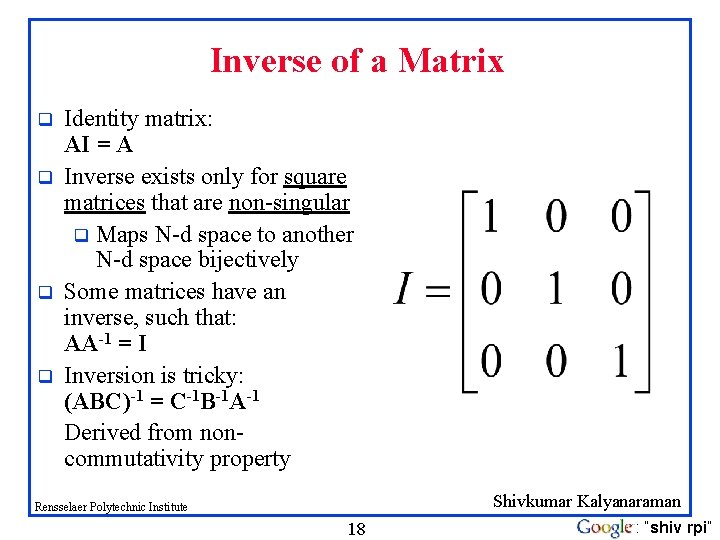Inverse of a Matrix q q Identity matrix: AI = A Inverse exists only for square matrices that are non-singular q Maps N-d space to another N-d space bijectively Some matrices have an inverse, such that: AA-1 = I Inversion is tricky: (ABC)-1 = C-1 B-1 A-1 Derived from noncommutativity property Shivkumar Kalyanaraman Rensselaer Polytechnic Institute 18 : “shiv rpi”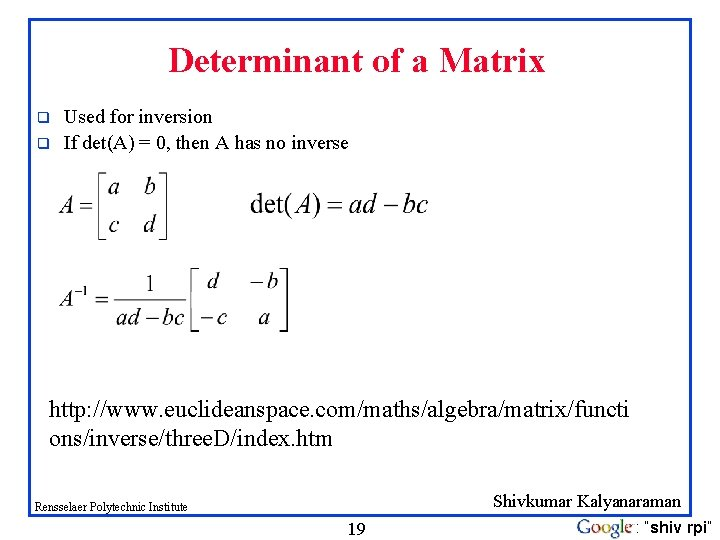Determinant of a Matrix q q Used for inversion If det(A) = 0, then A has no inverse http: //www. euclideanspace. com/maths/algebra/matrix/functi ons/inverse/three. D/index. htm Shivkumar Kalyanaraman Rensselaer Polytechnic Institute 19 : “shiv rpi”Projection: Using Inner Products (I) p = a (a. Tx) ||a|| = a. Ta = 1 Rensselaer Polytechnic Institute 20 Shivkumar Kalyanaraman : “shiv rpi”Homogeneous Coordinates Represent coordinates as (x, y, h) q Actual coordinates drawn will be (x/h, y/h) q Shivkumar Kalyanaraman Rensselaer Polytechnic Institute 21 : “shiv rpi”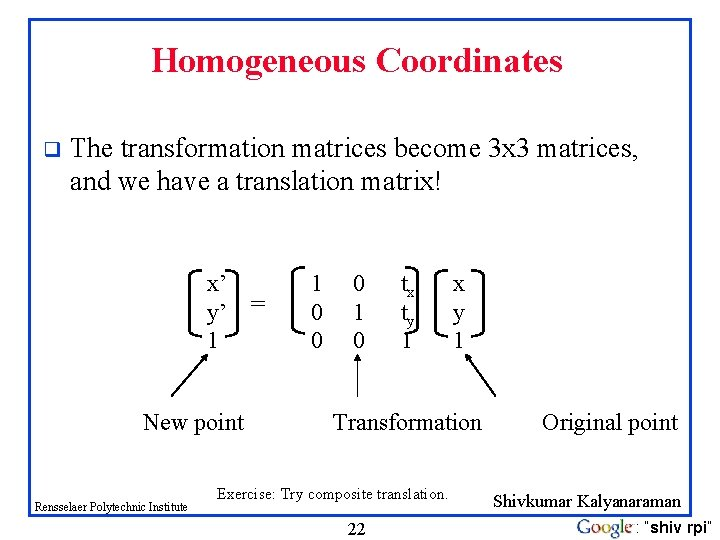Homogeneous Coordinates q The transformation matrices become 3 x 3 matrices, and we have a translation matrix! x’ y’ = 1 New point Rensselaer Polytechnic Institute 1 0 0 0 1 0 tx ty 1 x y 1 Transformation Exercise: Try composite translation. 22 Original point Shivkumar Kalyanaraman : “shiv rpi”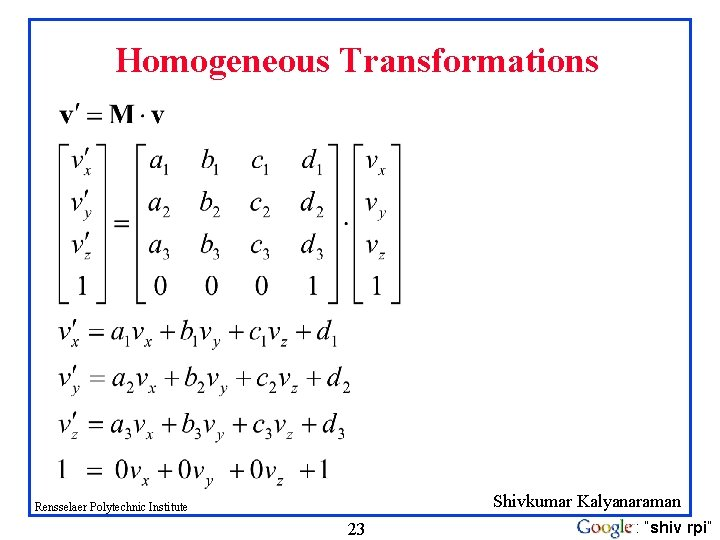Homogeneous Transformations Shivkumar Kalyanaraman Rensselaer Polytechnic Institute 23 : “shiv rpi”Order of Transformations Note that matrix on the right is the first applied q Mathematically, the following are equivalent p’ = ABCp = A(B(Cp)) q Note many references use column matrices to represent points. In terms of column matrices p’T = p. TCTBTAT q T R M Rensselaer Polytechnic Institute 24 Shivkumar Kalyanaraman 2 4 : “shiv rpi”Rotation About a Fixed Point other than the Origin Move fixed point to origin Rotate Move fixed point back M = T(pf) R( ) T(-pf) Rensselaer Polytechnic Institute 25 Shivkumar Kalyanaraman 2 5 : “shiv rpi”Vectors: Cross Product q The cross product of vectors A and B is a vector C which is perpendicular to A and B q The magnitude of C is proportional to the sin of the angle between A and B q The direction of C follows the right hand rule if we are working in a right-handed coordinate system A×B B A Shivkumar Kalyanaraman Rensselaer Polytechnic Institute 26 : “shiv rpi”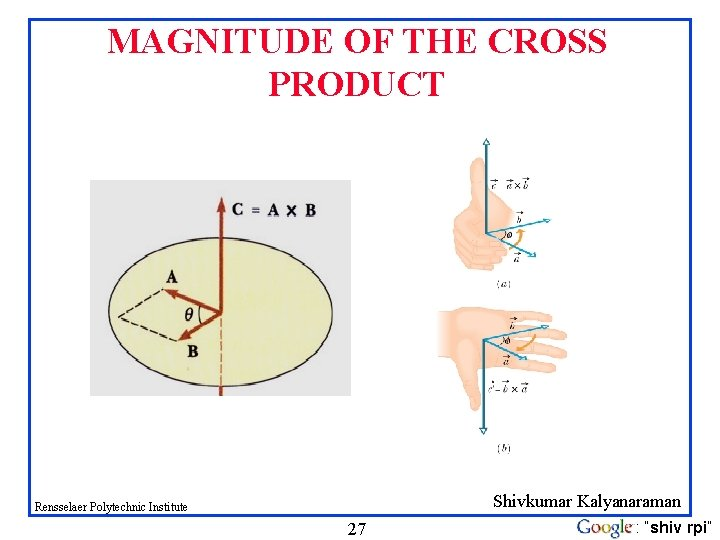MAGNITUDE OF THE CROSS PRODUCT Shivkumar Kalyanaraman Rensselaer Polytechnic Institute 27 : “shiv rpi”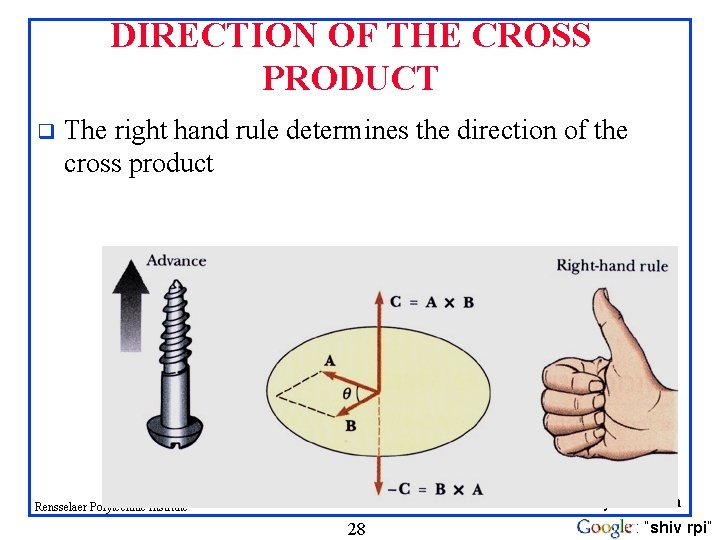DIRECTION OF THE CROSS PRODUCT q The right hand rule determines the direction of the cross product Shivkumar Kalyanaraman Rensselaer Polytechnic Institute 28 : “shiv rpi”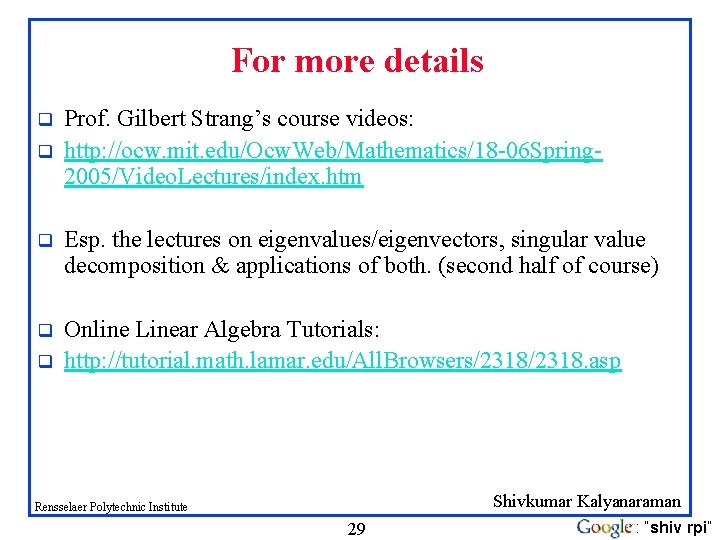For more details q q Prof. Gilbert Strang’s course videos: http: //ocw. mit. edu/Ocw. Web/Mathematics/18 -06 Spring 2005/Video. Lectures/index. htm q Esp. the lectures on eigenvalues/eigenvectors, singular value decomposition & applications of both. (second half of course) q Online Linear Algebra Tutorials: http: //tutorial. math. lamar. edu/All. Browsers/2318. asp q Shivkumar Kalyanaraman Rensselaer Polytechnic Institute 29 : “shiv rpi”